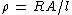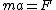# kinetics

(redirected from urea kinetics)
Also found in: Dictionary, Thesaurus, Medical.

## kinetics:

see dynamicsdynamics,
branch of mechanics that deals with the motion of objects; it may be further divided into kinematics, the study of motion without regard to the forces producing it, and kinetics, the study of the forces that produce or change motion.
.

## Kinetics (classical mechanics)

That part of classical mechanics which deals with the relation between the motions of material bodies and the forces acting upon them. It is synonymous with dynamics of material bodies. See Dynamics

Kinetics proceeds by adopting certain intuitively acceptable concepts which are associated with measurable quantities. These essential concepts and the measurable quantities used for their specification are as follows:

1. Space configuration refers to the positions and orientations of bodies in a reference frame adopted by the observer. It is expressed quantitatively by an arbitrarily chosen set of space coordinates, of which cartesian and polar coordinates are examples. All space coordinates rest on the notion of distance measurement.

2. Duration is expressed quantitatively by time measured by a clock or comparable mechanism.

3. Motion refers to change of configuration with time and is expressed by time rates of coordinate change called velocities and time rates of velocity change called accelerations. The classical assumption that coordinates behave as analytic functions of time permits representation of velocities and accelerations as first and second derivatives, respectively, of the space coordinates with respect to time.

4. Inertia is an attribute of bodies implying their capacity to resist changes of motion. A body's inertia with respect to linear motion is denoted by its mass.

5. Momentum is an attribute proportional to both the mass and velocity of a body. Momentum of linear motion is expressed as the product of mass and linear velocity.

6. Force serves to designate the influence exercised upon the motion of a particular body by other bodies, not necessarily specified. A quantitative connection between the motion of a body and the force applied to it is expressed by Newton's second law of motion, which is discussed later.

Distance, time, and mass are commonly regarded as fundamental, all other dynamical quantities being definable in terms of them.

A primary objective of classical kinetics is the prediction of the behavior of bodies which are subject to known forces when only initial values of the coordinates and momenta are available. This is accomplished by use of a principle first recognized by Isaac Newton. Newton's statement of the principle was restricted to the linear motion of an idealized body called a mass particle, having negligible extension in space.

The basic dynamical law set forth by Newton and known as his second law states that the time rate of change of a particle's linear momentum is proportional to and in the direction of the force applied to the particle. Stated analytically, Newton's second law becomes the differential equation (1),

(1)in which m represents the particle's mass, v its velocity, F the applied force, and t the time. Equation (1) provides a definition of force and of its units if units of mass, distance, and time have previously been adopted. The classical assumption of constancy of mass permits Eq. (1) to be expressed as Eq. (2),
(2)where a represents the linear acceleration.

The behavior of systems composed of two or more interacting particles is treated by Newtonian dynamics augmented by Newton's third law of motion which states that when two bodies interact, the forces they exert on one another are equal and oppositely directed. The important laws of momentum and energy conservation are derivable for such systems (the latter only for forces of special type) and useful in solution of problems. See Acceleration, Force, Gravitation, Harmonic motion, Mass, Momentum, Rigid-body dynamics, Velocity

## kinetics

[kə′ned·iks]
(mechanics)
The dynamics of material bodies.

## kinetics

1. another name for dynamics
2. the branch of mechanics, including both dynamics and kinematics, concerned with the study of bodies in motion
3. the branch of dynamics that excludes the study of bodies at rest
4. the branch of chemistry concerned with the rates of chemical reactions
Site: Follow: Share:
Open / Close